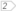#Boost C++ Libraries

...one of the most highly regarded and expertly designed C++ library projects in the world.

This is the documentation for a snapshot of the develop branch, built from commit 2f77332407.

### MultiIndex to Bimap Path - Bidirectional Map

This is example 4 in Boost.MultiIndex documentation.

This example shows how to construct a bidirectional map with multi_index_container. By a bidirectional map we mean a container of elements of `std::pair<const FromType,const ToType>` such that no two elements exists with the same first or second value (`std::map` only guarantees uniqueness of the first member). Fast look-up is provided for both keys. The program features a tiny Spanish-English dictionary with on-line query of words in both languages.

##### Boost.MultiIndex

```#include <iostream>
#include <boost/tokenizer.hpp>

#include <boost/multi_index_container.hpp>
#include <boost/multi_index/key_extractors.hpp>
#include <boost/multi_index/ordered_index.hpp>

using namespace boost;
using namespace boost::multi_index;

// tags for accessing both sides of a bidirectional map

struct from {};
struct to   {};

// The class template bidirectional_map wraps the specification
// of a bidirectional map based on multi_index_container.

template<typename FromType,typename ToType>
struct bidirectional_map
{
typedef std::pair<FromType,ToType> value_type;

typedef multi_index_container<
value_type,
indexed_by
<
ordered_unique
<
tag<from>, member<value_type,FromType,&value_type::first>
>,
ordered_unique
<
tag<to>, member<value_type,ToType,&value_type::second>
>
>

> type;

};

// A dictionary is a bidirectional map from strings to strings

typedef bidirectional_map<std::string,std::string>::type dictionary;

int main()
{
dictionary d;

// Fill up our microdictionary.
// first members Spanish, second members English.

d.insert(dictionary::value_type("hola","hello"));
d.insert(dictionary::value_type("rosa","rose"));
d.insert(dictionary::value_type("mesa","table"));

std::cout << "enter a word" << std::endl;
std::string word;
std::getline(std::cin,word);

// search the queried word on the from index (Spanish)

dictionary::iterator it = d.get<from>().find(word);

if( it != d.end() )
{
// the second part of the element is the equivalent in English

std::cout << word << " is said "
<< it->second << " in English" << std::endl;
}
else
{

dictionary::index_iterator<to>::type it2 = d.get<to>().find(word);
if( it2 != d.get<to>().end() )
{
std::cout << word << " is said "
<< it2->first << " in Spanish" << std::endl;
}
else
{
std::cout << "No such word in the dictionary" << std::endl;
}
}

return 0;
}
```

##### Boost.Bimap

```#include <iostream>
#include <boost/tokenizer.hpp>
#include <boost/bimap/bimap.hpp>

using namespace boost::bimaps;

// A dictionary is a bidirectional map from strings to strings

typedef bimap<std::string,std::string> dictionary;
typedef dictionary::value_type translation;

int main()
{
dictionary d;

// Fill up our microdictionary.
// first members Spanish, second members English.

d.insert( translation("hola" ,"hello"  ));
d.insert( translation("rosa" ,"rose"   ));
d.insert( translation("mesa" ,"table"  ));

std::cout << "enter a word" << std::endl;
std::string word;
std::getline(std::cin,word);

// search the queried word on the from index (Spanish)

dictionary::left_const_iterator it = d.left.find(word);

if( it != d.left.end() )
{
// the second part of the element is the equivalent in English

std::cout << word << " is said "
<< it->second<< " in English" << std::endl;
}
else
{

dictionary::right_const_iterator it2 = d.right.find(word);
if( it2 != d.right.end() )
{
std::cout << word << " is said "
<< it2->second<< " in Spanish" << std::endl;
}
else
{
std::cout << "No such word in the dictionary" << std::endl;
}
}

return 0;
}
```

 `it` is an iterator of the left view, so `it->second` refers to the right element of the relation, the word in english `it2` is an iterator of the right view, so `it2->second` refers to the left element of the relation, the word in spanish

Or better, using tags...

```#include <iostream>

#include <boost/bimap/bimap.hpp>

using namespace boost::bimaps;

// tags

struct spanish {};
struct english {};

// A dictionary is a bidirectional map from strings to strings

typedef bimap
<
tagged< std::string,spanish >, tagged< std::string,english >

> dictionary;

typedef dictionary::value_type translation;

int main()
{
dictionary d;

// Fill up our microdictionary.
// first members Spanish, second members English.

d.insert( translation("hola" ,"hello"  ));
d.insert( translation("rosa" ,"rose"   ));
d.insert( translation("mesa" ,"table"  ));

std::cout << "enter a word" << std::endl;
std::string word;
std::getline(std::cin,word);

// search the queried word on the from index (Spanish) */

dictionary::map_by<spanish>::const_iterator it =
d.by<spanish>().find(word);

if( it != d.by<spanish>().end() )
{
std::cout << word << " is said "
<< it->get<english>() << " in English" << std::endl;
}
else
{

dictionary::map_by<english>::const_iterator it2 =
d.by<english>().find(word);

if( it2 != d.by<english>().end() )
{
std::cout << word << " is said "
<< it2->get<spanish>() << " in Spanish" << std::endl;
}
else
{
std::cout << "No such word in the dictionary" << std::endl;
}
}

return 0;
}
```